# ECMAScript Functions Tutorial

## 1- What is Function?

In ECMAScript, A function means a subprogram, which includes commands to perform a duty. And when you want to do such duty somewhere in the application, you only need to call this function. The function helps you maintain the application and reuse code easily.
Below  is the syntax for defining a simple function without parameters:
```
function function_name() {
// function body
}

```
For example:
my-first-function-example.js
```
// Define a function:
function greeting()  {
console.log("Hello World!");
}

// Call function.
greeting();
```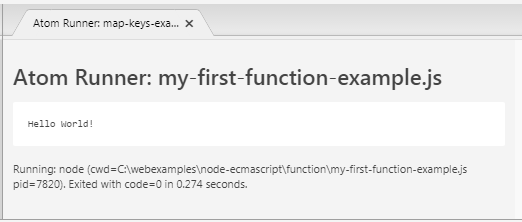A standard function in the ECMAScript is defined by the following syntax:
```function function_name(parameter1, parameter2, .., parameterN) {

// Function body
// return ....
}
```
• Use the function keyword to define a function.
• Functions can have 0 or many parameters.
• The function always returns a value, if this value is not indicated, it will return underfined. To return a value we use the return keyword.
standard-function-example.js
```
function calculateTax(salary)  {

if(salary >= 1000) {
// The function will end here
return salary * 20 / 100;
}
if(salary >= 500)  {
// The function will end here
return salary * 10 / 100;
}
return 0;
}

let tax = calculateTax(2000);
console.log("Salary: 2000, tax: " + tax);

tax = calculateTax(800);
console.log("Salary: 800, tax: " + tax);

tax = calculateTax(300);
console.log("Salary: 300, tax: " + tax);
```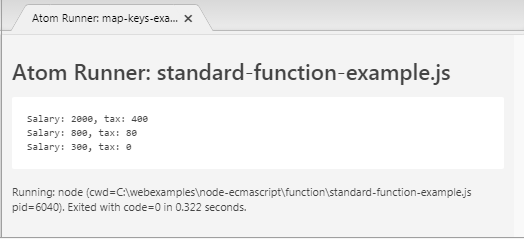## 2- Function with default parameter

In ES6, a function allows parameters to be initialized with default values. These default values will be used if you do not pass values to these parameters. (Or pass an undefined value when calling a function like the following example:
default-params-example1.js
```
function add(a, b = 1) {
return a+b;
}

// Test

console.log(value); // 5

// Test

console.log(value); // 10
```
default-params-example2.js
```
function calculateTax(salary = 1000, rate = 0.2) {
return salary * rate;
}

// Test
console.log (calculateTax() ) ; // 1000 * 0.2 = 200

console.log (calculateTax(2000) ) ; // 2000 * 0.2 = 400

console.log (calculateTax(3000, 0.5) ) ; // 3000 * 0.5 = 1500

console.log (calculateTax(undefined, 0.5) ) ; // 1000 * 0.5 = 500
```

## 3- Varargs parameter

Varargs parameter (Or also refered to as  "Rest Parameters" means a special parameter of a function. You can pass 0, 1 or more values to the  Varargs parameters.
characteristics of  Varargs parameter:
• If a function has a Varargs parameter, this parameter has to be the last parameter of the function.
• A function has at maximum 1 Varargs parameter.
```
function myFunction(param1, param2, ... paramVarargs)  {

}
```
varargs-param-example.js
```
function showInfo(name, ... points)  {
console.log("------------ ");
console.log("Name: " + name);

console.log("Array length: "+ points.length);

for(let i=0; i< points.length; i++)  {
console.log( points[i] );
}
}

showInfo("Tom", 52, 82, 93);

showInfo("Jerry", 10, 50);

showInfo("Donald");
```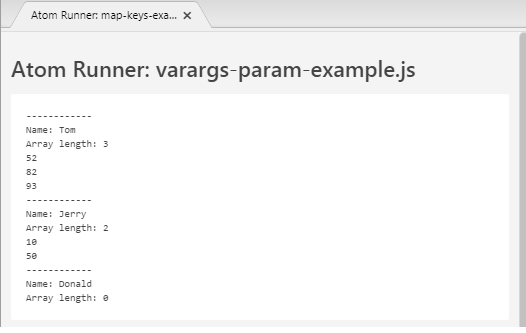## 4- Anonymous Function

A function having no name is called an anonymous function. Anonymous functions are dynamically created at runtime of the program. It also has parameters and returns like a common function.
You can also declare a variable with value as an anonymous function. Such an expression is called Function Expression.
```
var myVar = function( parameters ) {
// ....
}
```
Example:
anonymos-function-example.js
```
// Function expression
var f = function(name){
return "Hello " + name;
}

// Call it.
var value = f("Tom");

console.log( value ); // Hello Tom.
```
For example, create an anonymous function and call it immediately. In this case the anonymous function is created for only one use.
anonymous-function-example2.js
```
// Create anonymous function and call immediately
(function()  {
var now = new Date();

console.log("Now is " + now);
}) () ;

// Create anonymous function and call immediately
(function(name)  {

console.log("Hello " + name);
}) ("Tom") ;

```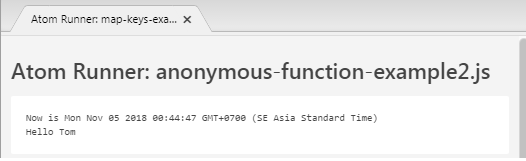## 5- Function Constructor

In the  ECMAScript, We have a Function class, so you can create a function through the constructors of this class.
```var variableName = new Function(paramName1, paramName2, ..., paramNameN, "Function Body");
```
For example:
function-constructor-example.js
```
var myMultiple = new Function( "x", "y", "return x * y");

console.log( myMultiple (3, 4) ); // 12

function showTotal()  {
let quantity = 4;
let price = 5;

console.log("Total: " + myMultiple(quantity, price) );
}

showTotal();
```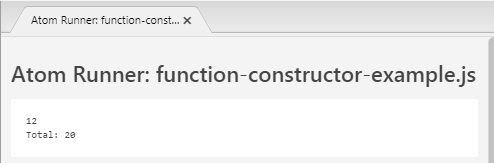## 6-  Lambda (Arrow) function

The Lambda function is actually an anonymous function written by a brief syntax. This function is also called " Arrow Function" because there is an arrow (=>) in its syntax.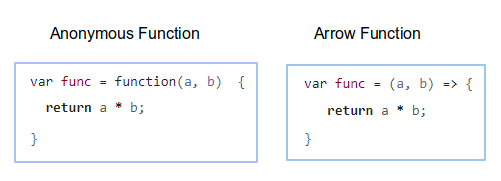Below is an anonymous function:
Anonymous Function
```// Anonymous Function
var func = function(a, b)  {
return a * b;
}
```
Also the above function but we write shorter by the  Lambda syntax.
```// Arrow function
var func = (a, b) => {
return a * b;
}

console.log( func( 5, 6 ) ); // 30
```
If the function has no parameters:
```// Function without parameter.
var func  =  () =>  {
console.log("Hello World");
}

console.log( func( ) ); // Hello World
```
If the function has only one parameter, you don't probably need to write the parentheses ():
```// Function with one parameter.
var func  = name =>  {
console.log("Hello " + name);
}

console.log( func( "Tom" ) ); // Hello Tom
```
If the function body  has only one expression, you don't probably  need to write brackets {} and remove the return keyword.
```// Body has only one expression.
var func = (name)  => {

console.log("Hello " + name)
}

// Body has only one expression.
var func  = (name) =>  console.log("Hello " + name);

```
```// Body has only one expression.
var func = (a, b)  => {

return a * b;
}

// Body has only one expression.
var func  = (a, b) =>  a * b;
```
For a function having one parameter, and the function body has only one expression which you can write the most concisely like the following example:
```
var func = (name)  => {
console.log("Hello " + name);
}

// Shortest:
var func = name => console.log("Hello " + name);
```

## 7- Generator Function

Generator Function is a new feature introduced into  ECMAScript 6 (ES6). To declare a Function Generator we use the function* keyword.
```
function* functionName( param1, param2, ..., paramN) {
// Statements
}
```
Basically,  Generator Function means a function that is able to suspend execution, and continue running at any time. The Function Generator returns an Iterator object in an implicit manner. In the contents of this function you only need to define the values for the elements in such  iterator.
Maybe you will feel really confused with the above explanation. We will see an example: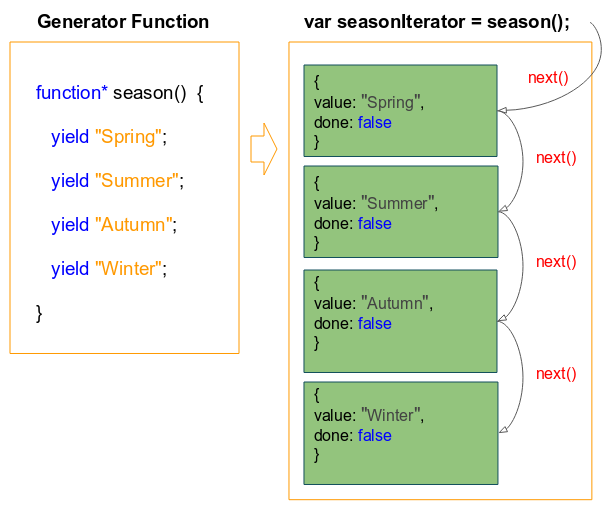generator-function-example.js
```
function* season()  {
yield "Spring";
yield "Summer";
yield "Autumn";
yield "Winter";
}

// This method return an Iterator.
var seasonIterator = season();

let value1 = seasonIterator.next().value;
console.log(value1); // Spring

let value2 = seasonIterator.next().value;
console.log(value2); // Summer

let value3 = seasonIterator.next().value;
console.log(value3); // Autumn

let value4 = seasonIterator.next().value;
console.log(value4); // Winter
```
Shorter example:
```
function* season()  {
yield "Spring";
yield "Summer";
yield "Autumn";
yield "Winter";
}

// This method return an Iterator.
var seasonIterator = season();

let e;
while( !(e = seasonIterator.next()).done ) {
console.log(e.value);
}
```
When a common function is called, it will execute until the value is returned or the function finishes. During that time the Caller Function can not control the execution of the Called Function.
Caller Function can control the execution of the  Called Function if the Called Function is a  Generator Function. Below is such example:
generator-function-example3.js
```
function* season()  {
console.log("Do something in Spring");
yield "Spring";// Spring Stop here!

console.log("Do something in Summer");
yield "Summer";// Summer Stop here!

console.log("Do something in Autumn");
yield "Autumn";// Autumn Stop here!

console.log("Do something in Winter");
yield "Winter";// Winter Stop here!
}

// This method returns an Iterator.
var seasonIterator = season();

let e;
while( !(e = seasonIterator.next()).done ) {
console.log(e.value);
if(e.value === "Autumn") {
console.log("OK Stop after Autumn");
break;
}
}
```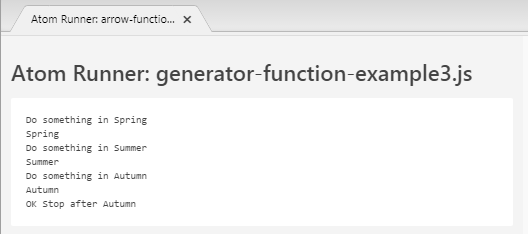#### View more Tutorials:

Maybe you are interested

These are online courses outside the o7planning website that we introduced, which may include free or discounted courses.

•Learning ECMAScript 6: Moving to the New JavaScript
•Master ExpressJS to Build Web Apps with NodeJS&JavaScript
•HTML5, CSS3 And JavaScript Fundamentals 2016
•Starting with JavaScript Unit Testing
•JavaScript Intro to learning JavaScript web programming
•Essentials of JavaScript Practice Coding Exercises Tips
•The Complete JavaScript Course - Beginner to Professional
•JavaScript in 55 Minutes
•2D Game Development With HTML5 Canvas, JS - Tic Tac Toe Game
•*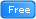* Introductory To JavaScript - Learn The Basics of JavaScript
•Become a Highly Paid JavaScript Developer in 2 Weeks
•Kids Coding - Introduction to HTML, CSS and JavaScript!
•Learn JavaScript Fundamentals
•Quick JavaScript Core learning Course JavaScript Essentials
•Javascript for Beginners